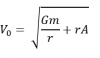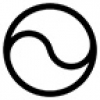## Hypersphere CosmologyAlternative to big-bang

Wednesday, 02 July 2014 16:10

## Ur Chaos Theory

In this paper 'Chaos' denotes randomness and indeterminacy, not the mere so-called 'deterministic chaos' of systems with extreme sensitivity to initial conditions.

For many decades it has seemed from theoretical considerations that true Chaos or indeterminacy applies only at the Planck length scale of ~10^-35 metres or the Planck time scale of ~10^-44 seconds. This hardly accords with either life as we experience it or what we know about the behaviour of fundamental particles or our own minds.

Evidence mounts for a substantial upward revision of the level at which Chaos actually shapes the behaviour of the universe and everything within it, including ourselves.

This paper considers the emerging holographic universe principle in terms of vorticitating hypersphere cosmology, (VHC).

The Beckenstein-Hawking conjecture asserts a proportionality between the surface area of a gravitationally closed structure like a black hole or a hypersphere, and its internal entropy.

The Claude Shannon information theory relates information to entropy.

Combined, these theories lead to the idea of a Holographic Universe in which each Planck area of the surface of the universe carries one bit of information. These bits of information serve to define what happens in all the Planck volumes within the universe.

This results in far less than one bit of information for each Planck volume within the universe. A holographic universe thus contains a substantial information deficit, and thus it behaves with more degrees of freedom, or more chaotically than simple quantum theories suggest.

In VHC the calculation of the ratio becomes quite simple. L, the antipode length of the universe has the value lp times U, where lp equals the Planck length, ~ 10^-35m, and U represents the huge number ~ 10^60. (U also relates the time and mass of the universe to the corresponding Planck quantities).

Thus if Vu represents the volume of the universe and Vp represents Planck volume then
Vu / Vp divided by Au / Ap = U
where Au equals the surface area of the universe and Ap equals the Planck area.

Thus the ratio of bits to Planck volumes comes out at only 1 bit per 10^60 Planck volumes.

This may seem a contra-intuitively small figure, however if we take the cube root of this volume figure to reduce it to a length measurement then it comes out at 10^20 Planck lengths. As the Planck length equals ~10^-35m this results in a figure of ~10^-15 metres, merely down at the fundamental particle level.

The information content of the universe cannot then specify its conditions down to the Planck scale, but only down to the scale of ~10^-15 metres, or the corresponding time of just ~10^-24 seconds.

Now we do not actually observe any real quantities below these levels anyway. Although fundamental particles theoretically exist as dimensionless points they have effective sizes down at the 10^-15m size when they interact, and similarly nothing ever seems to happen in less than 10^-24 seconds. These lower limits seem to represent the actual pixelation level of the universe, its 'grain size', and any real event either occupies a whole pixel or it doesn't happen. This minimum level of divisibility represents the point where causality ceases to apply and processes become indeterminate and Chaotic. Thus it's a whole lot more chaotic than Heisenberg thought.

This suggests that we should add the factor of the cube root of U to all of the Heisenberg uncertainty (indeterminacy) relationships, as in the following examples where D stands for Delta, the uncertainty, and h stands for slash-aitch, Planck's constant over 2 pi.

Momentum and Position. Dm Dp ~ h becomes Dm Dp ~ h (U)^1/3
and
Energy and time. DE Dt ~ h becomes DE Dt ~ h (U)^1/3
and also to the esoteric Entropy times temperature (SK) and time t, uncertainties which then becomes
DSK Dt ~ h (U)^1/3

This gives considerably more scope for the local spontaneous reversal of entropy that may allow the emergence of more complex forms from simpler ones.

The factoring in of cube root U seems to bring the indeterminacy of world up towards the scale at which we actually experience it, and it has two other consequences: -

Firstly it answers all those sarcastic physicists who opine that quantum based magical and mystical ideas remain invalid because quantum effects occur at a level far below the scale of brain cells for example:

Typical nerve conduction energy equates to~10^-15 joules and a typical nerve transmission time between neurones takes ~10^-3 seconds.

Applying this to DE Dt ~h (U)^1/3 suggests that Ur-Chaos enhanced quantum effects will certainly come into play at a brain cell level corresponding to actual thinking, the activation of about 1,000 neurones per second.

Thus Sir Roger Penrose's attempt, (in The Emperor's New Mind), to hypothesize such effects merely at the intracellular microtubule level perhaps seem rather too conservative now.

Secondly the value of U depends on the size of the universe. Bigger universes have a lower ratio of surface area to volume than smaller ones and hence behave more chaotically, so the value of U will affect the physical laws of the universe. A bigger universe will for example have fundamental particles with larger effective sizes, thus changing chemistry and radiation. When we examine 'fossil' light from distant galaxies or even the fossil or geological record of our own fairly old planet it appears that the laws of physics have remained essentially unchanged.

Thus if we inhabit a universe subject to the holographic principle it cannot have expanded.

So welcome to the wonderful world of Ur-Chaos, where everything has twenty more orders of magnitude of Chaos than previous calculations suggested, but we suspected something like this all along.

The title Ur-Chaos Theory derives from two mnemonics, as it suggests both U-root(3) and Uber or 'greater' Chaos.

An afterthought. In a lyrical sort of way, one might note that the bigger one's universe, i.e. the wider one's horizons, the more chaotic and interesting one's life becomes.

More in this category: « Quantum & Probability
• #### HyperSphere Cosmology +Hypersphere Cosmology in a Nutshell   1a) The Universe consists of a Hypersphere (a 3-Sphere or 4-ball) where: -       also
• #### Hypersphere Redshift +The alternative mechanism for redshift works as follows: Redshift = Z =   - 1 Where = observed wavelength, = expected wavelength, and the -1
• #### Hypersphere Rotation +The Gödel 3d rotating universe has this solution derived from General Relativity: - “Matter everywhere rotates relative to the compass of
• #### Hypersphere Holometry - A Prediction +As a consequence of the hypothesis that the universe exists as a hypersphere where   the main parameters of the
• #### VHC. Vorticitating Hypersphere Cosmology +The overwhelming majority of university and state funded cosmologists believe that the observable universe has expanded from a much smaller
• #### Hypersphere Visualisation & Lensing +This paper provides a method of visualising the four dimensional hypersphere as a perspective construction in three dimensions, and it
• #### Sacred Geometry +

As faith began to make way for reason during the Enlightenment, humans began to deduce the relationships between certain mathematical
• #### Starships +

Houston we have a problem. If the human race does not develop starships that can reach other star systems as
• #### Quantum & Probability +

I have repeatedly asserted in many of my magical and scientific books and papers that this universe runs on probability
• #### Ur Chaos Theory +

In this paper 'Chaos' denotes randomness and indeterminacy, not the mere so-called 'deterministic chaos' of systems with extreme sensitivity to
• 1